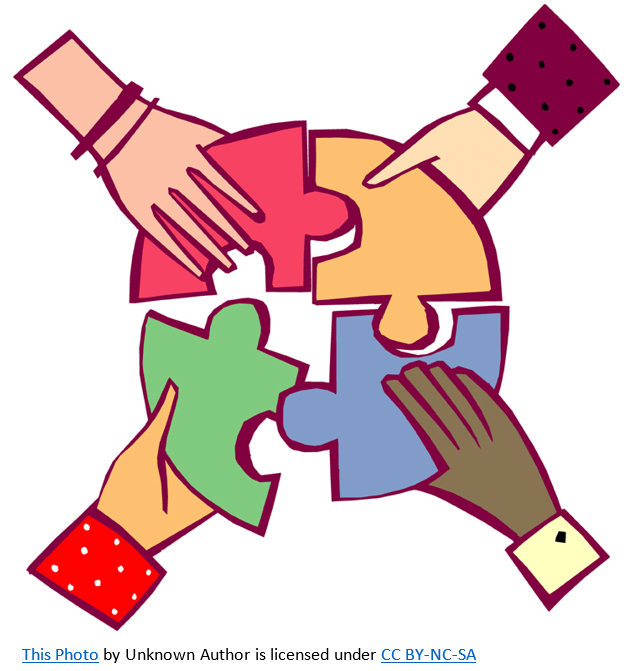# GEOM 3 | Lesson 4 | PracticeDraw a figure that represents the situation and solve.

1. Briana is using a mirror and a measuring tape to find the height of a building.  The mirror is placed 20′ from the base of the building.  Her eye height is $$5’6″$$.  She walked  $$5’$$ from mirror until she saw the top of the building in the mirror.  Find the height of the building.
2. Suzanne is also using a mirror and a measuring tape to find the height of the library.  Her eye height is $$5’3″$$.  The mirror is placed 20′ from the library.  She is $$1.75’$$ from the mirror when she looks in the mirror and sees the top of the library.   Find the height of the library.
3. The flagpole casts a $$15’$$ shadow.  At the same time Carlos, $$6’3″$$, casts a 3′ shadow.  Find the height of the flagpole.
4. As a tennis player you want to place the ball so that your opponent may have a hard time reaching it.  You want to place it 12′ on the other side of the net and the net is $$3.5’$$ high. Your generally hit the ball at a height $$8’$$ so how far from the net should you be when you hit the ball?
5. At noon, your $$6’3″$$ friend casts a $$2’$$ whereas a tree casts a $$9’$$ shadow.  How tall is the tree?

##### Check solutions here.